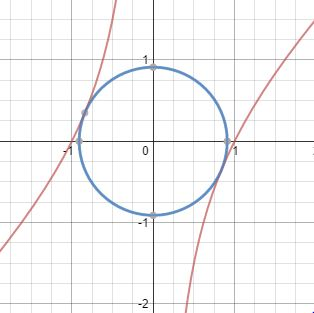# Connect the curve 2

Calculus Level 4The figure shows the graph of $f(x)=x-\frac{1}{x}$.

The circle is tangent to the graph in domain $x<0$ and $x>0$, and is the smallest of its kind.

The area of the circle is in the form $A\pi \left( -B+\sqrt { C } \right)$ where $A,B,C$ are positive integers with $C$ square free.

Find $A+B+C$.

###### More problems like this.
×

Problem Loading...

Note Loading...

Set Loading...